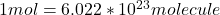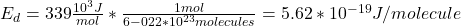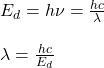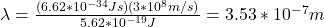## The c-cl bond dissociation energy in cf3cl is 339 kj/mol. What is the maximum wavelength of photons that can rupture this bond?

Question

The c-cl bond dissociation energy in cf3cl is 339 kj/mol. What is the maximum wavelength of photons that can rupture this bond?

in progress 0
2 months 2021-07-25T22:03:30+00:00 2 Answers 0 views 0

3.53*10^-7 color white “m

Where h= Planck constant

C=speed of light

Explanation:

Calculating energy required to dissociate the cl–cl Bond as this

Energy= +339*color white*kJmol

E=(339*10^3/6.022*10^23)=5.63*10^-19color white

But note from Planck equation

E=hf=h*c/lamda

Lamda= hc/E

=6.63*10^-34*3*10^8/5.63*10^-19

Lamda=3.532*10^-7 colorwhite*m

3.53*10^{-7} m

Explanation:

Photon that can rupture the bonds are those with the energy of the bond dissociation energy. If we want to know the energy for each molecule we have to take into account that:Hence, we havebut the energy is also:where h is the Planck’s constant and c is the speed of ligth. By replacing we obtain:hope this helps!